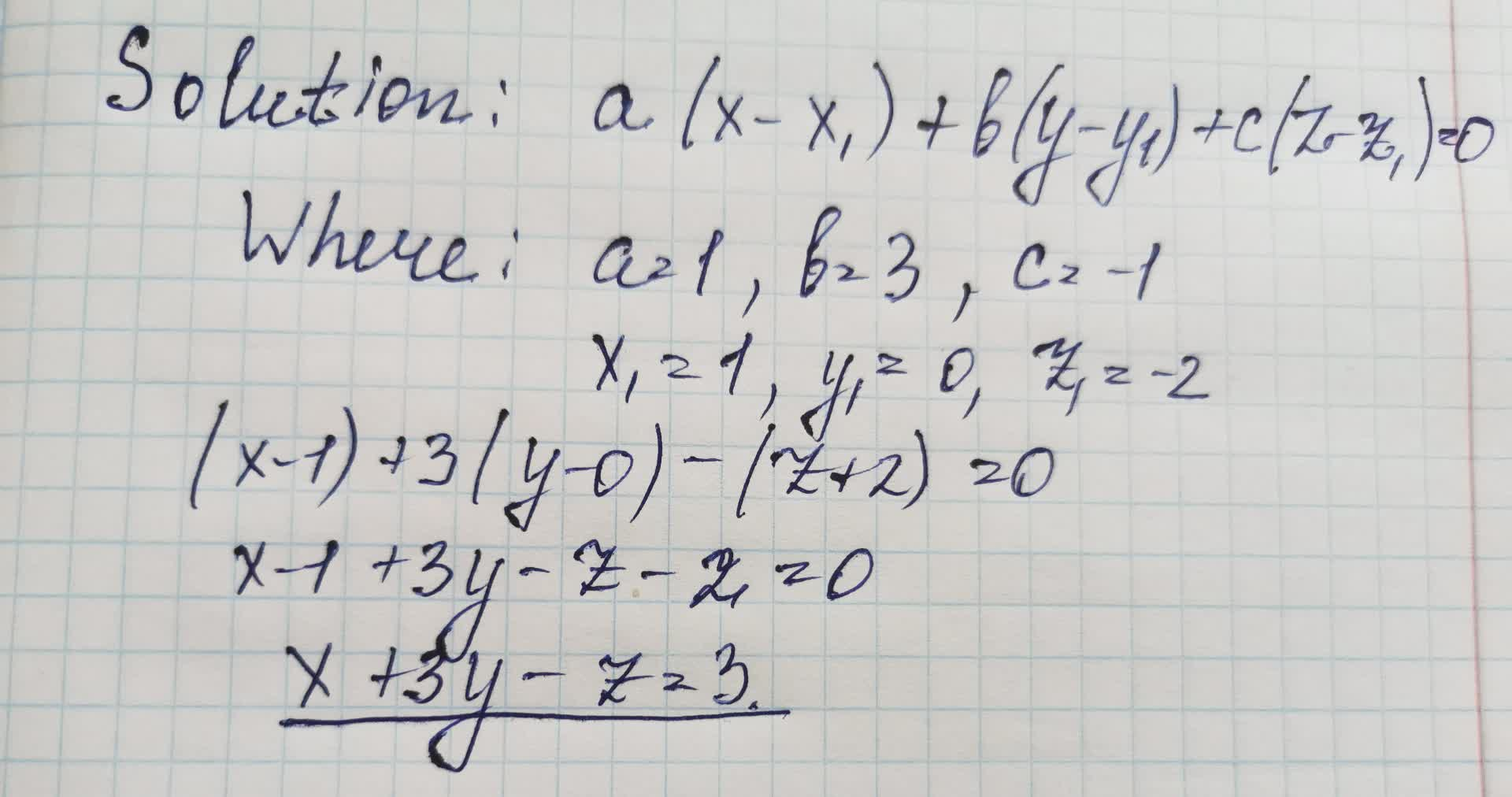Write the scalar equation for a plane through the point P_{0}=(1,0,-2) with normal vYasmin 2021-08-30 Answered

Write the scalar equation for a plane through the point $$\displaystyle{P}_{{{0}}}={\left({1},{0},-{2}\right)}$$ with normal vector $$v=(1,3,-1)$$

• Questions are typically answered in as fast as 30 minutes

Solve your problem for the price of one coffee

• Math expert for every subject
• Pay only if we can solve itdiskusje5

Scalar equation for a plane is written below